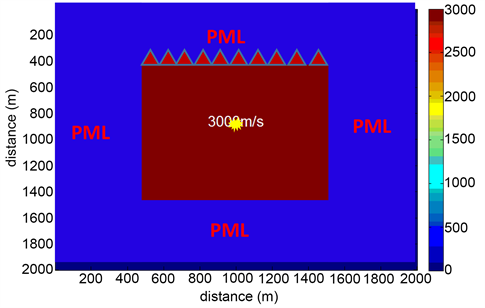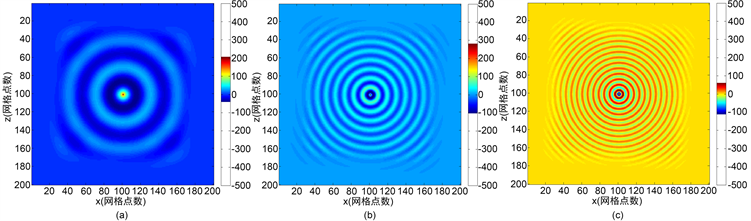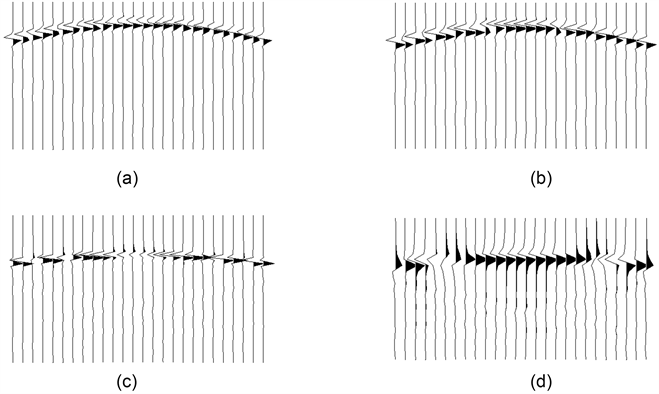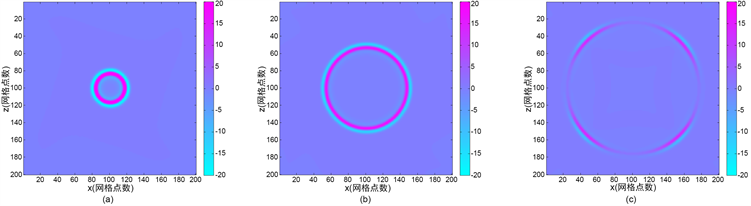﻿ 二维频率域声波方程正演模拟

# 二维频率域声波方程正演模拟2D Acoustic Wave Equation Forward Modeling in the Frequency Domain

Abstract: Forward modeling in frequency domain plays an important role in the numerical simulation of seismic waves. Compared with time domain forward modeling, frequency domain forward modeling has many advantages, such as suitable multi shot parallel operation, no time dispersion, flexible frequency band selection and small error. The coefficient matrix of different frequencies is relatively independent in the frequency domain forward modeling, which is suitable for the acceleration of parallel computing and greatly improves the computing efficiency. In this paper, for the optimal 9-point difference scheme of frequency domain acoustic equation, the implicit expression and sparse matrix solution are studied, and the seismic wave field is simulated forward. The accuracy and validity of the method are verified by model calculation.

1. 概述

Lysmer和Drake用有限元方法实现了地震波的数值模拟，初次提出了频率域正演模拟 。Marfurt指出频率域模拟地震波，能够非常有效地模拟出各向异性介质中波的传播及其衰减效应，而且适合大量炮点的同时模拟 。Pratt和Worthington将频率域正演引入到波形反演中，应用常规二阶差分法(5点有限差分格式)对声波方程进行了离散化处理，结果表明当每个波长取至少13个网格点时，相速度误差就可以控制在1%以内，研究过程中详细推导了频率域声波方程和弹性波方程差分格式，构建了阻抗矩阵，指出频率域正演模拟适合多炮计算(每个频率成分共用一个阻抗矩阵)，便于模拟衰减效应，为频率域波场正演奠定了基础 。由于频散严重和巨大的计算量等问题，频率域波场正演模拟没有得到广泛地应用，Jo和Shin等人在研究频率空间域声波波场正演模拟过程中，首次引入旋转坐标算子，将常规网格需要的5个网格点扩充到9个网格点，实现了频率域粘滞声波的正演模拟，频散得到了很好的压制，每个波长只需求4个网格就可以得到1%的精度要求，这使得频率域正演得到了极大地发展 。Shin在Jo的基础上，由9点差分格式扩充到25点，相对提高了精度，这样在正演过程中单个波长内的网格点可以减少到2.5个，得到了理想的结果 。

2. 频率域声波方程

$\frac{{\partial }^{2}u\left(x,z,t\right)}{\partial {x}^{2}}+\frac{{\partial }^{2}u\left(x,z,t\right)}{\partial {z}^{2}}-\frac{1}{v{\left(x,z\right)}^{2}}\frac{{\partial }^{2}u\left(x,z,t\right)}{\partial {t}^{2}}=-f\left(x,z,t\right)$

$\frac{{\partial }^{2}u\left(x,z,\omega \right)}{\partial {x}^{2}}+\frac{{\partial }^{2}u\left(x,y,\omega \right)}{\partial {z}^{2}}-\frac{{\omega }^{2}}{{v}^{2}}u\left(x,z,\omega \right)=-f\left(x,z,\omega \right)$

3. 波动方程正演模拟

4. 边界条件

5. 模型计算Figure 1. Velocity model of homogeneous mediumFigure 2. Real part of frequency slice ((a)-(c) are 10 Hz, 30 Hz and 50 Hz real part slices)Figure 3. Single shot records in time domain ((a)-(d) are time domain single shot record after 400, 300, 200, 100 frequency components inverse transformation)Figure 4. Time slice ((a)-(c) are 0.10 s, 0.20 s and 0.30 s time slice)

6. 结论

1) 频率切片的实部和虚部从低频到高频变化时，同心圆逐渐加密；

2) 在同频率成分下实部和虚部幅值大小不同；

3) 反傅里叶变换时，频率越多时间域精度越高，但是频率成分超过震源子波有值区域后，即使频率成分增加，精度也不会有太大变化。

NOTES

*通讯作者。

 Lysmer, J. and Drake, L. (1972) A Finite Element Method for Seismology. In: Alder, B., Fernbach, S. and Bolt, B.A., Eds., Methods in Computational Physics, Vol. 11, Academic Press, New York.

 Marfurt, K.J. (1984) Accuracy of Finite-Difference and Finite-Element Modeling of the Scalar and Elastic Wave Equations. Geophysics, 49, 533-549.
https://doi.org/10.1190/1.1441689

 Pratt, R.G. and Worthington, M.H. (1990) Inverse Theory Applied to Multi-Source Cross-Hole Tomography. Part 1: Acoustic Wave-Equation Method. Geothysical Prospecting, 38, 287-310.
https://doi.org/10.1111/j.1365-2478.1990.tb01846.x

 Jo, C.H., Shin, C. and Suh, J.H. (1996) An Optimal 9-Point, Finite-Difference, Frequency Space, 2D Scalar Wave Extrapolator. Geophysics, 61, 529-537.
https://doi.org/10.1190/1.1443979

 Shin, C., Yoon, K., Marfurt, K.J., Park, K., Yang, D., Lim, H., Chung, S. and Shin, S. (2001) Efficient Calculation of a Partial Derivative Wavefield Using Reciprocity for Seismic Imaging and Inversion. Geophysics, 66, 1856-1863.
https://doi.org/10.1190/1.1487129

 Min, D.J., Yoo, H.S., Shin, C., et al. (2000) Improved Frequency-Domain Elastic Wave Modeling Using Weighted- Averaging Difference Oprators. Geophysics, 65, 884-895.
https://doi.org/10.1190/1.1444785

 Hustedt, B., Operto, S. and Virieux, J. (2004) Mixed-Grid and Stag-gered-Grid Finite-Difference Methods for Frequency-Domain Acoustic Wave Modeling. Geophysical Journal Interna-tional, 157, 1269-1296.
https://doi.org/10.1111/j.1365-246X.2004.02289.x

 Xu, K., Zhou, B. and McMechan, G.A. (2010) Imple-mentation of Prestack Reverse Time Migration Using Frequency-Domain Extrapolation. Geophysics, 75, 61-72.
https://doi.org/10.1190/1.3339386

 曹书红, 陈景波. 声波方程频率域高精度正演的17点格式及数值实现[J]. 地球物理学报, 2012, 55(2): 3440-3449.

 刘璐, 刘洪，刘红伟. 优化15点频率–空间域有限差分正演模拟[J]. 地球物理学报, 2014, 56(2): 644-652.

 卞爱飞, 於文辉, 周华伟. 频率域全波形反演方法研究进展[J]. 地球物理学进展, 2010(3): 982-993.

 董良国, 迟本鑫, 陶纪霞, 刘玉柱. 声波全波形反演目标函数性态[J]. 地球物理学报, 2013, 56(10): 3445-3460.

 高凤霞, 刘财, 冯晅, 鹿琪, 王典. 几种优化方法在频率域全波形反演中的应用效果及对比分析研究[J]. 地球物理学进展, 2013(4), 2060-2068.

Top